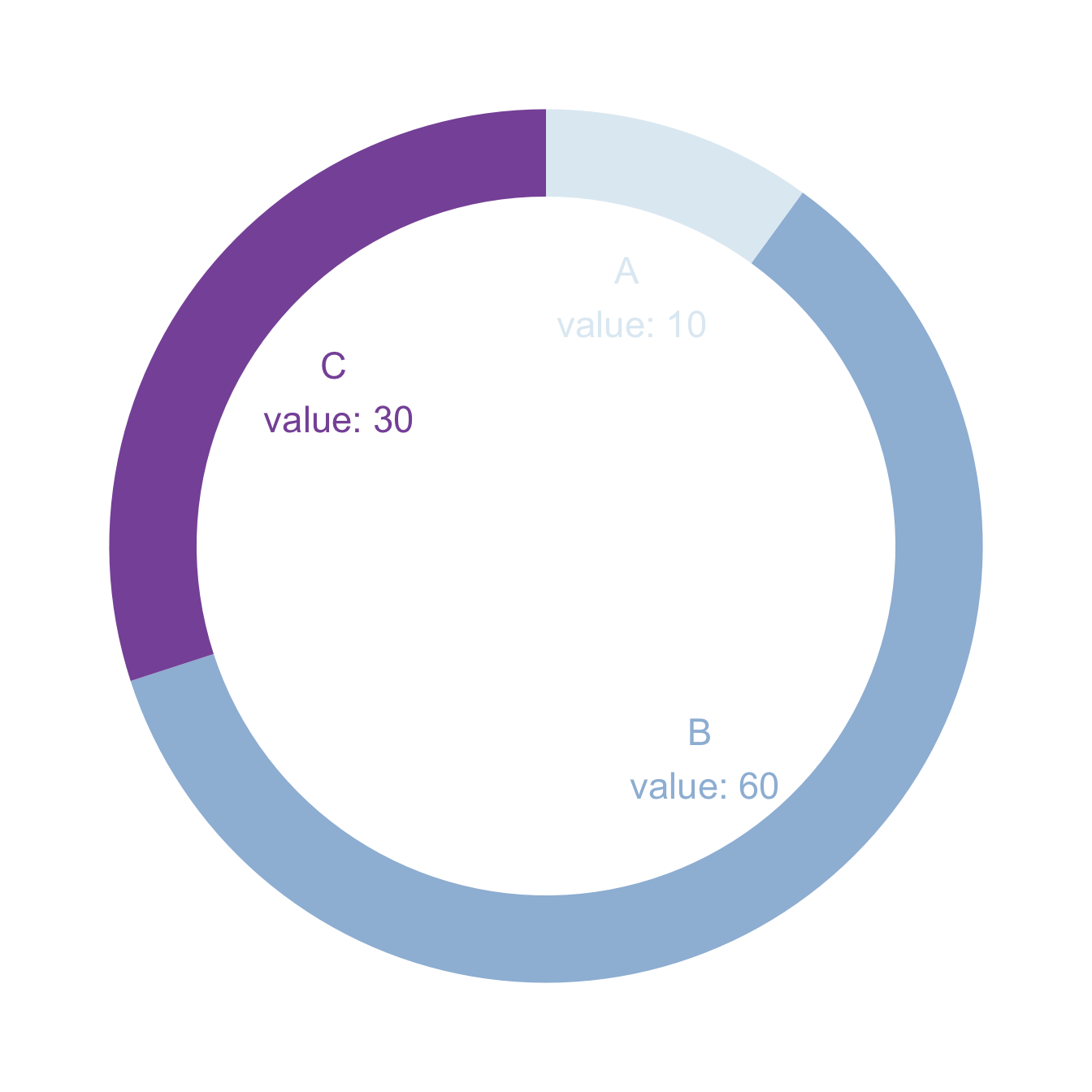# Donut chart with ggplot2

The ggplot2 package allows to build donut chart with R. This post describes how, providing explanation and reproducible code.

# Most basic doughnut chart with `ggplot2`

The `ggplot2` package allows to build donut charts. Note however that this is possible thanks a hack, since no specific function has been created for this kind of chart. (This is voluntary, to avoid donut charts that are dataviz bad practice)

Here is the process: - input data provides a numeric variable for a set of entities - absolute numeric values must be translated to proportion - group positions must be stacked: we’re gonna display them one after the other - `geom_rect()` is used to plot each group as a rectangle - `coord_polar()` is used to switch from stacked rectangles to a ring - `xlim()` allows to switch from pie to donut: it adds the empty circle in the middle``````# load library
library(ggplot2)

# Create test data.
data <- data.frame(
category=c("A", "B", "C"),
count=c(10, 60, 30)
)

# Compute percentages
data\$fraction = data\$count / sum(data\$count)

# Compute the cumulative percentages (top of each rectangle)
data\$ymax = cumsum(data\$fraction)

# Compute the bottom of each rectangle

# Make the plot
ggplot(data, aes(ymax=ymax, ymin=ymin, xmax=4, xmin=3, fill=category)) +
geom_rect() +
coord_polar(theta="y") + # Try to remove that to understand how the chart is built initially
xlim(c(2, 4)) # Try to remove that to see how to make a pie chart``````

# Customization

Here are a couple of things you can do improve your donut chart style:

• use `theme_void()` to get rid of the unnecessary background, axis, labels and so on.
• use a better color palette
• don’t use a legend, add labels to groups directly``````# load library
library(ggplot2)

# Create test data.
data <- data.frame(
category=c("A", "B", "C"),
count=c(10, 60, 30)
)

# Compute percentages
data\$fraction <- data\$count / sum(data\$count)

# Compute the cumulative percentages (top of each rectangle)
data\$ymax <- cumsum(data\$fraction)

# Compute the bottom of each rectangle

# Compute label position
data\$labelPosition <- (data\$ymax + data\$ymin) / 2

# Compute a good label
data\$label <- paste0(data\$category, "\n value: ", data\$count)

# Make the plot
ggplot(data, aes(ymax=ymax, ymin=ymin, xmax=4, xmin=3, fill=category)) +
geom_rect() +
geom_label( x=3.5, aes(y=labelPosition, label=label), size=6) +
scale_fill_brewer(palette=4) +
coord_polar(theta="y") +
xlim(c(2, 4)) +
theme_void() +
theme(legend.position = "none")``````

# Donut thickness

It is important to understand that donut chart are just stacked rectangles that are made circular thanks to `coord_polar`.

Thus, the empty circle that makes it a donut chart is just the space between the initial Y axis and the left part of the rectangle.

• If `xlim` left boundary is big, no empty circle. You get a pie chart
• If `xlim` is low, the ring becomes thinner.

If you don’t get it, just plot the chart without `coord_polar()```````# load library
library(ggplot2)

# Create test data.
data <- data.frame(
category=c("A", "B", "C"),
count=c(10, 60, 30)
)

# Compute percentages
data\$fraction <- data\$count / sum(data\$count)

# Compute the cumulative percentages (top of each rectangle)
data\$ymax <- cumsum(data\$fraction)

# Compute the bottom of each rectangle

# Compute label position
data\$labelPosition <- (data\$ymax + data\$ymin) / 2

# Compute a good label
data\$label <- paste0(data\$category, "\n value: ", data\$count)

# Make the plot
ggplot(data, aes(ymax=ymax, ymin=ymin, xmax=4, xmin=3, fill=category)) +
geom_rect() +
geom_text( x=2, aes(y=labelPosition, label=label, color=category), size=6) + # x here controls label position (inner / outer)
scale_fill_brewer(palette=3) +
scale_color_brewer(palette=3) +
coord_polar(theta="y") +
xlim(c(-1, 4)) +
theme_void() +
theme(legend.position = "none")``````

Related chart types

## Contact

This document is a work by Yan Holtz. Any feedback is highly encouraged. You can fill an issue on Github, drop me a message on Twitter, or send an email pasting yan.holtz.data with gmail.com.